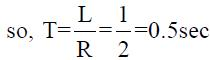Courses

# Network Theory

## 25 Questions MCQ Test GATE Electrical Engineering (EE) 2022 Mock Test Series | Network Theory

Description
This mock test of Network Theory for GATE helps you for every GATE entrance exam. This contains 25 Multiple Choice Questions for GATE Network Theory (mcq) to study with solutions a complete question bank. The solved questions answers in this Network Theory quiz give you a good mix of easy questions and tough questions. GATE students definitely take this Network Theory exercise for a better result in the exam. You can find other Network Theory extra questions, long questions & short questions for GATE on EduRev as well by searching above.
QUESTION: 1

Solution:
QUESTION: 2

Solution:
QUESTION: 3

### Two AC sources feed a common variable resistive load as shown in figure below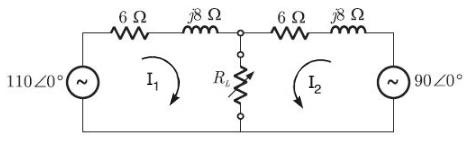The maximum power that can be transferred to a variable load RL is _______ W.

Solution: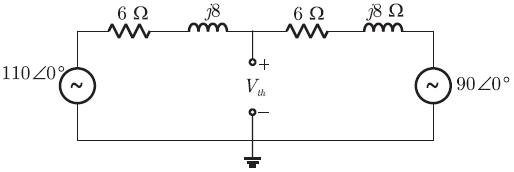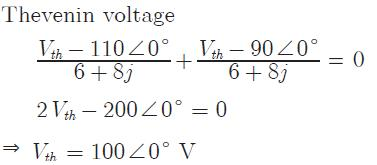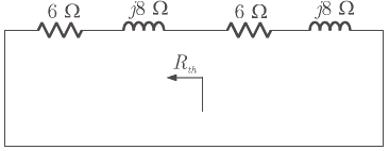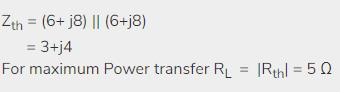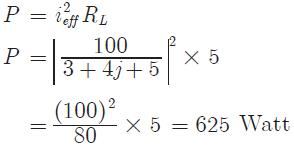QUESTION: 4

The switch of below circuit was open for long and at t = 0 it is closed. What is the final steady state voltage across the capacitor and the time constant of the circuit?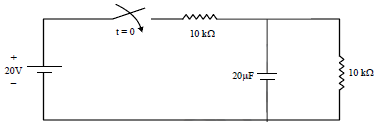Solution:

Final value = 20/2 = 10 Volt
Time constant τ= 5 × 103 × 20 × 10-6
= 0.1 sec

QUESTION: 5

The y parameter of the network given in figure.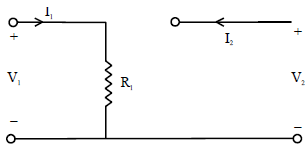Solution: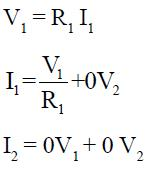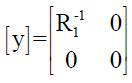QUESTION: 6

The power consumed by a coil is 300 W when connected to 30 V dc source and 108 W. When connected to a 30 V A.C. source. The reactance of the coil is

Solution:

In DC, inductive reactance X=0; since f=0;

Only, Resistance exist in coil for DC,

R=(30*30/300)=3 ohm;

In AC, Z=R+jX;

But here in second part of question we given that real power in ac circuit is 108 watt . We can calculate current in the ac circuit

I=√(108/3)= √36=6 amp

This current flow through resistance of AC circuit so this is the current of AC circuit.

So,

Z =V/I

Z=30/6=5

X=√{5^2–3^2}=4ohm

QUESTION: 7

For a transmission line open circuit and short circuit impedances are 20 Ω and 5 Ω. Then characteristic impedance is

Solution:
QUESTION: 8

A human nerve cell has an open circuit voltage of 80 mV and it can deliver a current of 5 mA through a 6 MΩ load. What is the maximum power available from the cell?

Solution: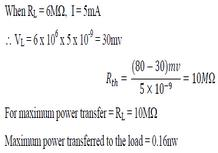QUESTION: 9

The Thenenin impedance across the terminal AB of the given network is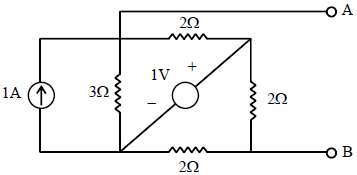Solution: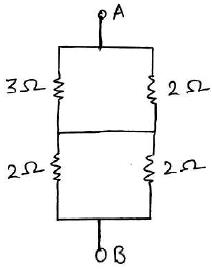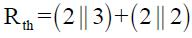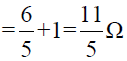QUESTION: 10

The circuit shown in the figure Ris variable between 3 and 30 ohms RL being fixed
Assertion (A): For Maximum power transfer across the terminals AB, Rg = 3?
Reason (R): When Maximum power transfer occurs RL = |Zg|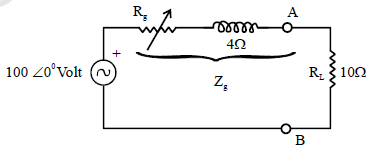Solution: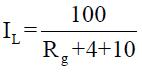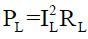PL is Maximum when IL is Maximum
IL is Maximum when Rg in Minimum = 3Ω
A is True
For Maximum power Transfer KL= |Zs|
But here Rg is variable, so it should be low so R is True but not the correct explanation of A.

QUESTION: 11

A line to neutral voltage is 10∠150V for a balanced three phase star connected load with phase sequence ABC. The voltage of line B with respect to line C is given by

Solution: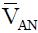= 10 ∠150 Volt
Phase sequence is ABC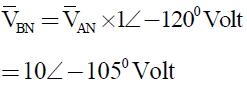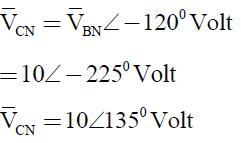Line Voltage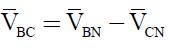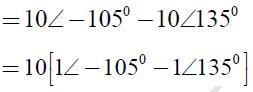= 10[1∠ - 1200 x 1∠15 - 1∠1200 x 1∠15]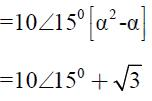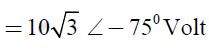*Answer can only contain numeric values
QUESTION: 12

Determine Maximum power consumed in the load resistance R_____ (in watts)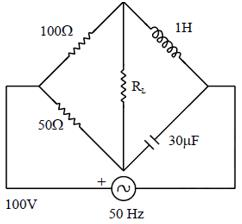(Important - Enter only the numerical value in the answer)

Solution:

ω = 2πf = 2π x 50 = 100π
XL = ωL , Xc = 1/ωc
Thevenin equivalent across RL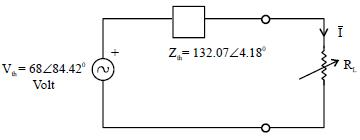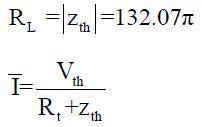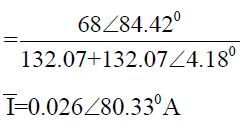PMax = ± 2RL = (0.026)2 x 132.07
PMax = 8.92 Watt

QUESTION: 13

A series R-L-C circuit resonates at 4Hz it provides 0.707 lag P.F. at 8 Hz. The frequency at which it gives 0.707 lead P.F is?

Solution:

f0 = 4 Hz,
Upper cut off frequency
f2 = 8Hz
lower cutoff f1 = ?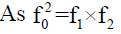(4)2 = f1 x 8
16 = f1 x 8
f1 = 2Hz

QUESTION: 14

In series RLC circuit, the voltage across capacitor and inductor are ______ with each other.

Solution:

In series RLC circuit, the voltage across capacitor and inductor are 180⁰ out of phase with each other. The frequency at which the resonance occurs is called resonant frequency.

QUESTION: 15

A series R-L-C circuit has a resonance frequency of 1kHz and a quality factor Q = 100. If each of R, L and C is doubled from its original value then new Q of the circuit is

Solution: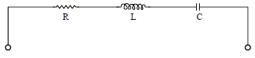Quality factor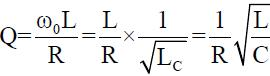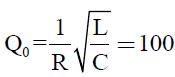if R, L, C double
R' = 2R, L' = 2L, C' = 2C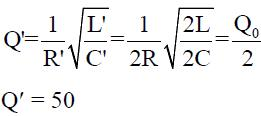*Answer can only contain numeric values
QUESTION: 16

The system is initially at rest for voltage signal of unit step i(t) = ?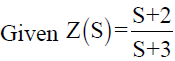(Important - Enter only the numerical value in the answer)

Solution: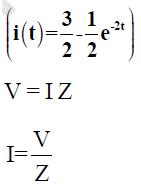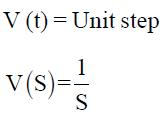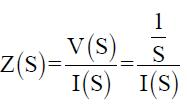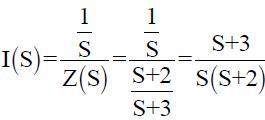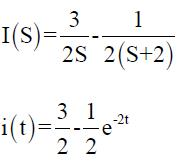QUESTION: 17

Consider the following function

1.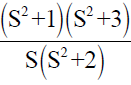2.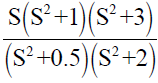3.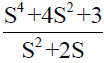4.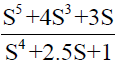Which of the above function are LC driving point impedances?

Solution:

Only (1) and (2) are L-C driving point impedance.

QUESTION: 18

If an RC driving point impedance function Z(S) has equal number of poles and zero’s at finite locations then

Solution:

Let us Consider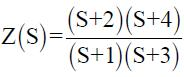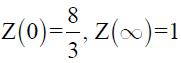QUESTION: 19

For the circuit shown below the pole’s of the driving point impedance function are at which one of the following location?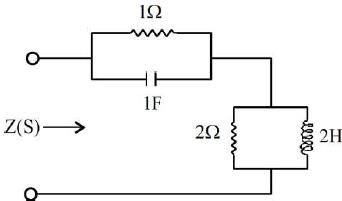Solution: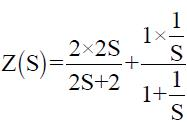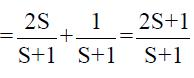A Pole at S = -1 and a zero at S = - 1/2.

QUESTION: 20

The voltage and current wave functions for an element are shown in the figures.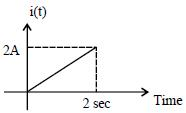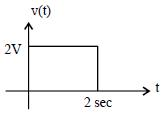The circuit element and its value are

Solution: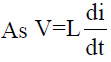For L = 2H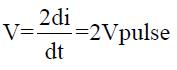So element is inductor and its value is 2H

QUESTION: 21

The steady state in the circuit shown in the given figure is reached with S open. S is closed at t = 0. The current I ar t = 0+ is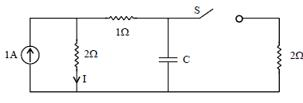Solution: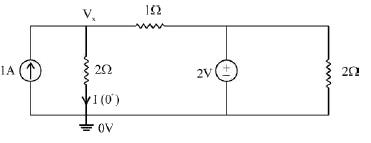KCL and node Vx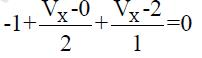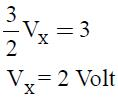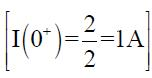QUESTION: 22

The total impedance Z(Jω) of the circuit shown below is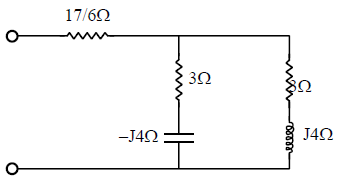Solution: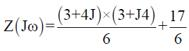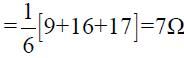QUESTION: 23

Identify which of the following is not a tree of the graph shown in figure.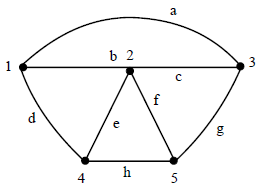Solution:

Tree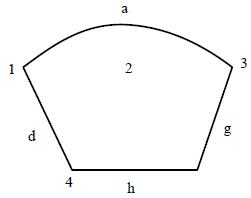QUESTION: 24

Two lamps each of 230V and 60W rating are connected in series across a single phase 230V supply. The total power consumed by the two lamps would be

Solution: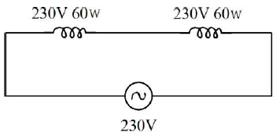Resistance of each lamp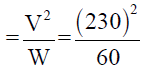Hence Total resistance offered by 2 these lamps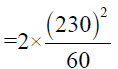So total power consumed by two lamps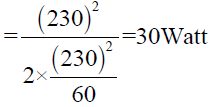QUESTION: 25

For the R-L circuit shown the current i(t) for unit step input voltage will rise to 0.63 is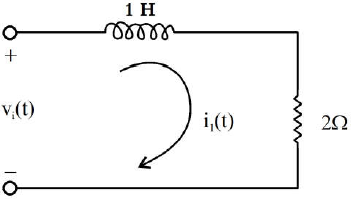Solution:

The time at which the current rise to 63% of its final value is Time constant,